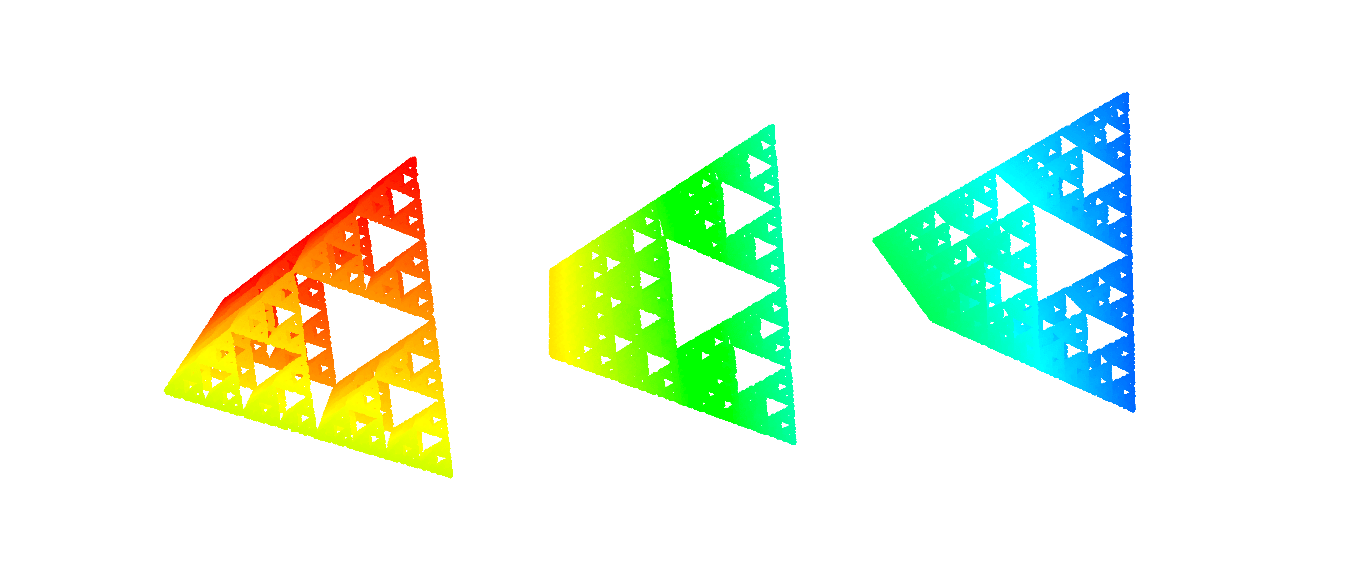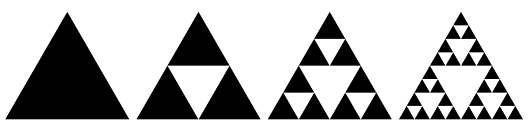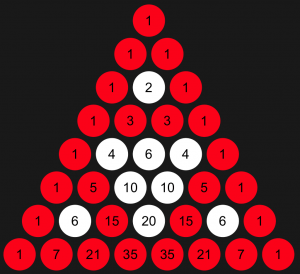# 100 years with the Sierpinski triangle

Three ways to obtain and generalise a beautiful fractal2015 is the 100th anniversary of the Sierpinski triangle, first described by Wacław Sierpiński, a Polish mathematician who published 724 papers and 50 books during his lifetime! The famous triangle is easily constructed by following these steps:

• Divide that triangle into four equilateral triangles and remove the one in the centre.
• Repeat the same steps with the remaining triangles, dividing each one into four triangles and removing the one in the centre.Construction of the Sierpinski triangle

A very neat thing of this simple procedure is that the result of doing the same process, over and over again, is a fractal. What does that mean? It looks the same no matter how much we zoom in. It’s a pattern that repeats inside itself.

Some mathematical properties of that fractal: If the first triangle has area $A$, then dividing the triangle into four parts and only keeping three of them gives a remaining area of

$$\left(\frac{3}{4}\right)A;$$

and following the same steps, over and over again, each time dividing the area into four parts and only keeping three of them, by the $n$-th step we are left with

$$\left( \frac{3}{4} \right)^n A.$$

This means, that each step has a smaller area than the one before and tends to zero if we carry on forever.

Now, if the initial triangle has a perimeter of say, $P$, then by the next step, the shaded area has a perimeter of

$$\left( \frac{3}{2}\right) P,$$

as we must add the perimeter of the triangle we have cut out. The perimeter grows at each step, and by the $n$-th step, it can be expressed as

$$\left( \frac{3}{2} \right)^n P.$$

So we have this very neat result: at each step we reduce the area, eventually to zero, and we increase the perimeter, which tends to infinity. In fact, if we start with a triangle that has a perimeter of only $1 cm$, then by following the procedure a mere 62 times, the perimeter of the shaded area would stretch to go to the moon and back to Earth, and everything inside that beautiful triangle would fit on the tip of your finger. By the 62nd step, the area of the shaded region would be only $3.45 \times 10^{-9}$cm2, invisible to the human eye, and impossible to display using the pixels of a computer screen, but certainly not beyond the scope of our imagination!

There are other ways to obtain the Sierpinski triangle. For example, if we begin with an array of numbers, where we put just a $’1’$ in the first row, two $’1’$s in the second row, and then, from the third row onwards we obtain the figure in each position simply by adding the two numbers directly above it, with a $’1’$ at both sides of each row, we would obtain the famous Pascal triangle. This triangle is very useful, since on the $k$-th row it tells us each of the coefficients that we need to write in the expansion of the polynomial $(x + y)^k$.

But what is the connection between this array of numbers and the Sierpinski triangle? If we have enough rows of the Pascal triangle and we highlight only the odd numbers, we discover our old friend: the Sierpinski triangle.Pascal triangle with odds and even numbers highlighted in a different colour

Awesome, right? Observing a fractal pattern, such as the Sierpinski triangle, within a simple array of odd and even numbers shows that the connections between different mathematical subjects can be found in the most unexpected places. But is that all? Of course not! Mathematics is about getting a result, and then taking it to its limits. Change something, see what you get and then keep going. The way we obtained the Sierpinski triangle starting from the Pascal triangle was by taking the rows and then dividing the numbers into odds and evens; or, equivalently, we divided each of the numbers by 2 and focused only on the residuals. Now, what happens if we focus on the residuals but we divide by a different number? If we divide by the number $k$ and we use a different colour for each of the possible residuals, we obtain a $k$-Sierpinski triangle. The following gallery shows the first 641 rows of different $k$-Sierpinski triangles.

This slideshow requires JavaScript.

The result is a generalised version of the Sierpinski triangle! And, something really stunning is to see all those beautiful patterns in the $k$-Sierpinski triangle that were hidden in the traditional version of the triangle, particularly when the number $k$ is prime.

A third way to get the  Sierpinski triangle is as follows. On a piece of paper draw three fixed points, $A$, $B$ and $C$, and then pick $A$ as the initial point $x_0$. Then, pick one of the three fixed points at random (which can easily be done by rolling a regular dice and then picking $A$ if the number that comes up is either 1 or 2, $B$ if the number is 3 or 4 and $C$ if it is 5 or 6), and then go from $x_0$ to the midpoint of the chosen point $A$, $B$ or $C$, and mark a new point, $x_1$. Then again pick any of the three fixed points $A$, $B$ or $C$ and move to the midpoint between that one and $x_1$, marking a new point $x_2$, and so on. At each step you move from the last $x_k$ to the midpoint between $x_k$ and one of the points $A$, $B$ or $C$, which have been picked at random.

Maybe after the first steps it is hard to see the pattern that will emerge, since there are just a few points in pretty random positions, but do it enough times and you begin to see the Sierpinski triangle, with the points $A$, $B$ and $C$ as its vertices. Amazing, right? Even when you move in a random and very unpredictable pattern, the same hollow triangle keeps emerging.

This slideshow requires JavaScript.

If we plot not only the marks $x_0, x_1, \dots$, but we also plot the line that connects every two consecutive points, the result is a beautiful figure that could be drawn without taking your pen off the paper.

And is that all? Again, no! Mathematicians, we like to push things to the limit. What happens if instead of fixing only three points in a plane, we now fix four points in 3D space? If now we have $A$, $B$, $C$ and $D$ and we move randomly to the midpoint to any of those four points, we obtain a Sierpinski pyramid.

We could even pick $k$ points in a $k-1$ dimensional space and then, by moving randomly to the midpoints, we would obtain a generalised Sierpinski fractal, which now we would not be able to observe (because, I mean, how on Earth are we going to be able to visualise that many dimensions?!). A fractal that lives beyond the limitations of our eyes, but not beyond the limits of our imagination.Rafael Prieto Curiel is doing a PhD in mathematics and crime. He is interested in mathematical modelling of any social issues, such as road accidents, migration, crime, fear and gossip.